Home

# A Fractal Function Related to the John–Nirenberg Inequality for Qα(ℝn)

## Abstract

A borderline case function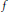$f$ for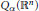${{Q}_{\alpha }}\left( {{\mathbb{R}}^{n}} \right)$ spaces is defined as a Haar wavelet decomposition, with the coefficients depending on a fixed parameter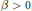$\beta \,>\,0$ . On its support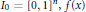${{I}_{0}}\,=\,{{\left[ 0,\,1 \right]}^{n}},\,f\left( x \right)$ can be expressed by the binary expansions of the coordinates of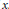$x$ . In particular,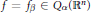$f\,=\,{{f}_{\beta }}\,\in \,{{Q}_{\alpha }}\left( {{\mathbb{R}}^{n}} \right)$ if and only if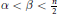$\alpha \,<\,\beta \,<\frac{n}{2}$ , while for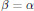$\beta \,=\,\alpha$ , it was shown by Yue and Dafni that$f$ satisfies a John–Nirenberg inequality for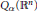${{Q}_{\alpha }}\left( {{\mathbb{R}}^{n}} \right)$ . When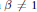$\beta \,\ne \,1$ ,$f$ is a self-affine function. It is continuous almost everywhere and discontinuous at all dyadic points inside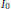${{I}_{0}}$ . In addition, it is not monotone along any coordinate direction in any small cube. When the parameter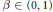$\beta \,\in \,\left( 0,\,1 \right)$ ,$f$ is onto from${{I}_{0}}$ to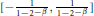$\left[ -\frac{1}{1-{{2}^{-\beta }}},\,\frac{1}{1-{{2}^{-\beta }}} \right]$ , and the graph of$f$ has a non-integer fractal dimension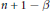$n\,+\,1\,-\beta$ .

• # Send article to Kindle

Note you can select to send to either the @free.kindle.com or @kindle.com variations. ‘@free.kindle.com’ emails are free but can only be sent to your device when it is connected to wi-fi. ‘@kindle.com’ emails can be delivered even when you are not connected to wi-fi, but note that service fees apply.

Find out more about the Kindle Personal Document Service.

A Fractal Function Related to the John–Nirenberg Inequality for Q α(ℝn)
Available formats
×

# Send article to Dropbox

To send this article to your Dropbox account, please select one or more formats and confirm that you agree to abide by our usage policies. If this is the first time you use this feature, you will be asked to authorise Cambridge Core to connect with your <service> account. Find out more about sending content to Dropbox.

A Fractal Function Related to the John–Nirenberg Inequality for Q α(ℝn)
Available formats
×

# Send article to Google Drive

To send this article to your Google Drive account, please select one or more formats and confirm that you agree to abide by our usage policies. If this is the first time you use this feature, you will be asked to authorise Cambridge Core to connect with your <service> account. Find out more about sending content to Google Drive.

A Fractal Function Related to the John–Nirenberg Inequality for Q α(ℝn)
Available formats
×

## Footnotes

Hide All

Supported in part by NSERC and the CRM, Montréal.

## References

Hide All
MathJax
MathJax is a JavaScript display engine for mathematics. For more information see http://www.mathjax.org.

# A Fractal Function Related to the John–Nirenberg Inequality for Qα(ℝn)

## Metrics

### Full text viewsFull text views reflects the number of PDF downloads, PDFs sent to Google Drive, Dropbox and Kindle and HTML full text views.

Total number of HTML views: 0
Total number of PDF views: 0 *Loading metrics...

### Abstract viewsAbstract views reflect the number of visits to the article landing page.

Total abstract views: 0 *Loading metrics...

* Views captured on Cambridge Core between <date>. This data will be updated every 24 hours.

Usage data cannot currently be displayed